# Crack IBPS Clerk Prelims 2018 – Sectional Full Test-3 | Numerical Ability

Dear Aspirants, IBPS Clerk 2018 Preliminary Examination is scheduled to be held on 8th, 9th, 15th and 16th of December. Candidates who have a dream to crack the IBPS Clerk 2018 need to speed up the preparation from now.

Our IBPS Guide team is providing a full-length Sectional Tests for English Language, Reasoning Ability and Numerical Ability with precise solutions for assisting you to crack IBPS Clerk Prelims exam.

Take Free Quantitative Aptitude Sectional Test of 35 Questions as like in the real exam to analyze your preparation level. Our Sectional Test Questions are taken as per the latest exam pattern and so it will be really useful for you to crack the prelims exam lucratively. Students who are weak in Reasoning Ability should utilize this chance constructively to accomplish a successful profession in Banking Field.

[WpProQuiz 4110]

Directions (Q. 1 – 5) Study the following table carefully and answer the given questions:

The following bar graph shows the total number of employees working in 3 different departments in 5 different organizations.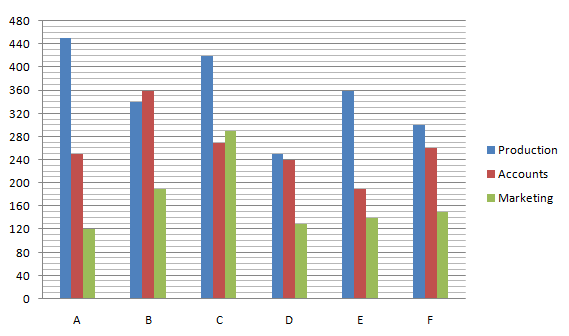1) Find the ratio between the total number of employees in all the given departments together in the organization B to that of D?

a) 34 : 19

b) 89 : 62

c) 15 : 7

d) 123 : 104

e) None of these

2) The total number of employees in all the given departments together in the organization A is approximately what percentage more/less than the total number of employees in all the given departments together in the organization C?

a) 16 % more

b) 25 % less

c) 25 % more

d) 16 % less

e) 37 % less

3) Find the difference between the total number of employees in Production department to that of Accounts department in all the given organization together?

a) 550

b) 620

c) 480

d) 710

e) None of these

4) Total employees in marketing department in the organization A, C and E together is what percentage of total employees in Accounts department in the organization B and D together?

a) 85.33 %

b) 78.28 %

c) 91.67 %

d) 96.52 %

e) None of these

5) Find the average number of employees in the marketing department in all the given organizations together?

a) 170

b) 185

c) 160

d) 200

e) None of these

Directions (6-10): What value should come in the place of question mark in the given questions?

6) 3.5, 33.5, 83.5, 153.5, 243.5, ?

a) 453.5

b) 353.5

c) 553.5

d) 293.5

e) 283.5

7) 21, 24, 29, 36, 47, ?

a) 60

b) 50

c) 65

d) 68

e) 54

8) 1400, 1481, 1564, 1649, 1736, ?

a) 1835

b) 1845

c) 1825

d) 1855

e) 1815

9) 66, 91, 120, 153, ?, 231

a) 190

b) 180

c) 200

d) 201

e) 167

10) 12167, 9261, 6859, ?, 3375, 2197

a) 4913

b) 4096

c) 5832

d) 4449

e) 1728

11) The vendor sells the 20 % of apple for Esai and he sells 80% of the reminder for Adhi. Still he has 40 dozen of apple, then find how many apples did he originally have?

a) 2000

b) 3400

c) 2500

d) 3000

e) None of these

12) In a 60 litres mixture of milk and water in a vessel, the ratio of milk and water is 3:1. Another vessel has milk and water in the ratio of 7:5. How many liters of the second mixture of milk must be poured into 60 litres of the first mixture so that the new mixture has milk and water in the ratio of 3:2?

a) 600 litres

b) 480 litres

c) 540 litres

d) 630 litres

e) None of these

13) The average age of students in the class is 18.75 years. 22 students newly join the class with an average age of 14.25 years. Now the class average becomes 16 years. Find the number of students in the class?

a) 32

b) 36

c) 30

d) 28

e) None of these

14) Subi, Pavin and Chinu started the business by investing the amounts of Rs.20000, Rs.40000 and Rs.10000 respectively. Subi invested the amount for 8 months, Pavin invested the amount for 12 months and Chinu invested the amount for 6 months only. If the lowest profit obtained by any of these three is Rs.1500, then what is the total annual profit obtained from the business?

a) Rs.16590

b) Rs.14500

c) Rs.15000

d) Rs.17500

e) None of these

15) If the length of the rectangle increased by 30% and the breadth of the rectangle is decreased by 20%. Then find the area will be increased or decreased by.

a) 4% increased

b) 3% decreased

c) 5% decreased

d) 2% increased

e) None of these

Directions (16-20): Study the following graph carefully and answer the given questions.

Number of people (in thousands) using six different types of mobile services in four different cities.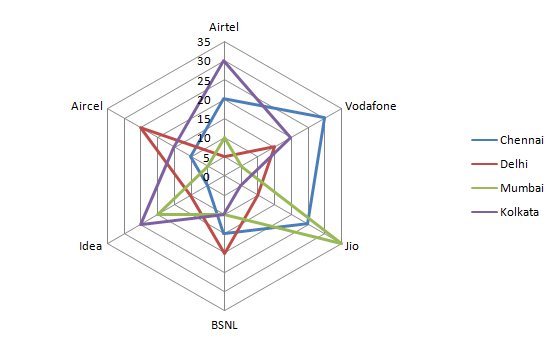16) The total number of people using mobile service Airtel and Vodafone for all the given cities is approximately what percentage more or less than the total number of people using mobile service in Delhi and Mumbai?

a) 20% less

b) 25% more

c) 15% less

d) 20% more

e) 30% less

17) Maximum number of people using in which of the following mobile service for all the given cities together?

a) Airtel

b) Vodafone

c) Jio

d) Idea

e) BSNL

18) What is the average number of people using the mobile service in Kolkata except Aircel?

a) 16500

b) 15000

c) 18000

d) 17500

e) None of these

19) Minimum number of people using the mobile service in which of the following city for all the given mobile service together?

a) Chennai

b) Delhi

c) Both Delhi and Mumbai

d) Both Kolkata and Mumbai

e) None of these

20) What is the ratio of the number of people using Idea mobile service in the entire city to that of the number of people using Aircel mobile service in the entire city?

a) 12:11

b) 13:12

d) 18:11

d) 14:13

e) None of these

Directions (21-25): What value should come in the place of question mark in the given questions?

21) 136% of 650-(3/5)*360+65% of 480=?

a) 880

b) 980

c) 780

d) 680

e) None of these

22) 160÷12.5*4.5+34.1*4+156.8*5=?

a) 878

b) 678

c) 978

d) 578

e) 888

23) 16% of 80+? % of 132=88.6-21.8+78

a) 100

b) 120

c) 150

d) 180

e) 50

24) 73% of 180+23% of 780+135% of 960=?

a) 1506.8

b) 1606.8

c) 1706.8

d) 1406.8

e) None of these

25) 14580÷54*12+1530÷17+456=?

a) 3786

b) 2786

c) 4786

d) 1786

e) None of these

26) If the price of sugar increased by 25% and Ovi can spend only 15% more than the current expenditure. Then by how much % Ovi needs to reduce the sugar consumption?

a) 6%

b) 8%

c) 5%

d) 4%

e) None of these

27) If 12 men and 16 women do a piece of work in 6 days and 6 men and 18 women do the piece of work in 8 days. How long days will 8 men and 24 women take to complete the same work?

a) 6 days

b) 12 days

c) 8 days

d) 7 days

e) None of these

28) A car covered a distance of 480km in 6 hours, covering a part of it at 60kmph and the remaining at 90kmph. For how much time the car travel at 90kmph?

a) 3 hours

b) 5 hours

c) 4 hours

d) 2 hours

e) None of these

29) The sum of two even numbers is 8 more than twice of smaller number. If the difference between these two numbers is 8, find the smaller number?

a) 6

b) 8

c) 10

d) 12

30) The simple interest on a certain sum at a certain annual rate of interest is 36% of the sum. If the rate of interest and the number of years is equal, then find the rate of interest is?

a) 10%

b) 8%

c) 6%

d) 12%

e) None of these

Directions (31-35): What is the approximate value should come in the place of question mark in the given questions?

31) (13.05)2-(4.98)2+(8.02)2-(5.045)3=?

a) 83

b) 80

c) 79

d) 88

e) 73

32) 2809÷27.99*5.25-125.08-246.19=?

a) 164

b) 138

c) 144

d) 129

e) 139

33) 806.01÷34.99*25.01+719.98*1.998=?

a) 2048

b) 2037

c) 2020

d) 2015

e) 2105

34) 23% of 435.03-(11/7) % of 1734.07+ (9/5)*890.01=?

a) 1675

b) 1575

c) 1775

d) 1875

e) 1795

35) (5741+3829+8374+124) ÷ (291+468+192) +131.20=?

a) 160

b) 150

c) 120

d) 110

e) 180

Direction (1-5) :

The total number of employees in all the given departments together in the organization B

= > 340 + 360 + 190 = 890

The total number of employees in all the given departments together in the organization D

= > 250 + 240 + 130 = 620

Required ratio = 890 : 620 = 89 : 62

The total number of employees in all the given departments together in the organization A

= > 450 + 250 + 120 = 820

The total number of employees in all the given departments together in the organization C

= > 420 + 270 + 290 = 980

Required % = [(980 – 820)/980]*100 = 16 % less

The total number of employees in Production department in all the given organization together

= > 450 + 340 + 420 + 250 + 360 + 300 = 2120

The total number of employees in Accounts department in all the given organization together

= > 250 + 360 + 270 + 240 + 190 + 260 = 1570

Required difference = 2120 – 1570 = 550

Total employees in marketing department in the organization A, C and E together

= > 120 + 290 + 140 = 550

Total employees in Accounts department in the organization B and D together

= > 360 + 240 = 600

Required % = (550/600)*100 = 91.67 %

The average number of employees in marketing department in all the given organizations together

= > (120 + 190 + 290 + 130 + 140 + 150)/6

= > 1020/6 = 170

Direction (6-10) :

3.5+30=33.5

33.5+50=83.5

83.5+70=153.5

153.5+90=243.5

243.5+110=353.5

21+3=24

24+5=29

29+7=36

36+11=47

47+13=60

1400+81=1481

1481+83=1564

1564+85=1649

1649+87=1736

1736+89=1825

66+25=91

91+29=120

120+33=153

153+37=190

190+41=231

233=12167

213=9261

193=6859

173=4913

153=3375

133=2197

Assume, initially vendor has 100x apples. He sells 20% for Esai, so the remaining are 80x apples.

He sells 80% of 80x apples for Adhi=64x

Then he has 16x remaining, so 16x=40 dozens

16x=40*12

x=30

So, initially the vendor have=100(30) = 3000 apples.

In the first vessel the volume of milk=60*3/4=45 and the water=60*1/4=15.

Second vessel ratio of milk and water=7:5.

X litres of second mixture be poured into the first mixture,

(45+7x/12) / (15+5x/12) =3/2

90+14x/12=45+15x/12

x/12=45

x=540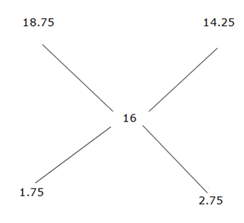Required ratio = 1.75: 2.75 = 175: 275

= 7: 11

The number of students in the class=18*22/11

=36

Ratio of profit = 20000*8:40000*12:10000*6

=16:48:6

=8:24:3

Chinu get the minimum share of the profit, hence the total annual profit

= (35/3)*1500

=17500

Let’s assume initial area=100

Area increased or decreased by=100*130/100*80/100

=104

=4% increased

Direction (16-20) :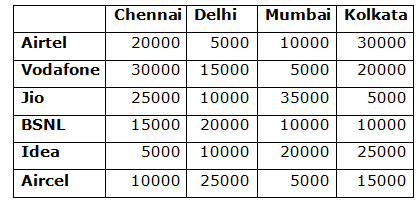Total number of people using the mobile service Vodafone=30000+15000+5000+20000

=70000

Total number of people using the mobile service Airtel=20000+5000+10000+30000

=65000

Vodafone + Airtel=70000+65000=135000

Total number of people using mobile service in Delhi=5000+15000+10000+20000+10000+25000

=85000

Total number of people using mobile service in Mumbai=10000+5000+35000+10000+20000+5000

=85000

Total Mumbai and Delhi=85000+85000=170000

Required percentage=100*(170000-135000)/170000

=20% less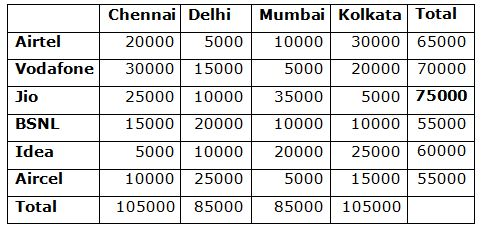From the above table, the maximum number of people using the mobile service is Jio.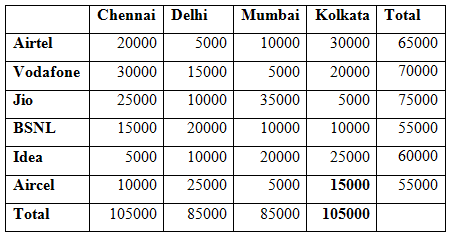Total number of people using the mobile service in Kolkata=105000 – 15000

= 90000

Average= (90000)/5 =18000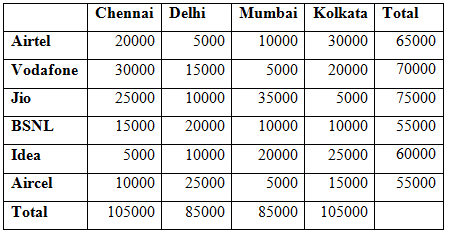From the above table, number of people using mobile service is minimum, both Delhi and Mumbai.Required ratio=60000:55000  = 12:11

136% of 650-(3/5)*360+65% of 480=?

884-216+312=?

980=?

160÷12.5*4.5+34.1*4+156.8*5=?

57.6+136.4+784=?

978=?

16% of 80+?% of 132=88.6-21.8+78

12.8+?% of 132=144.8

?%132=132

?=100

73% of 180+23% of 780+135% of 960=?

131.4+179.4+1296=?

1606.8=?

14580÷54*12+1530÷17+456=?

3240+90+456=?

3786=?

Reduce consumption by (125-115)*100/125=8%

(12M+16W)*6=(6M+18W)*8

36M+48W=24M+72W

12M=24W

1M=2W

M/W=2/1=2:1

From the ratio

(12*2+16*1)*6= (8*2+24*1)*x

40*6=40*x

x=6 days

Average speed=480/6=80kmph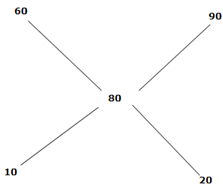Ratio=1:2

Actual time=6 hours

Required time=2*6/3

=4 hours

Let’s assume, Bigger number=X

Smaller number=Y

Sum of two even numbers is 8 more than twice of smaller number, X+Y=2Y+8

X-Y=8

Difference between the two numbers is 8, X-Y=8

We can’t find the exact value x and y from the equations. So the data provided are not adequate to the answer.

Sum=x

Simple interest=36x/100

Time and rate of interest is equal, so the rate R=SI*100/P*T

R=((36x/100)*100)/x*R

R2=36

R=6%

(13.05)2-(4.98)2+(8.02)2-(5.045)3=?

169-25+64-125=?

83=?

2809÷27.99*5.25-125.08-246.19=?

100*5 -371=?

129=?

806.01÷34.99*25.01+719.98*1.998=?

805/35 * 25 + 720 * 2 =?

23*25+1440=?

575 + 1440 =?

2015=?

23% of 435.03-(11/7) % of 1734.07+ (9/5)*890.01=?

100-27+1602=?

1675=?

(5741+3829+8374+124)÷ (291+468+192) +131.20=?

18068÷951+131.20=?

19+131=?

150=?